# sklearn.model_selection.train_test_split¶

sklearn.model_selection.train_test_split(*arrays, test_size=None, train_size=None, random_state=None, shuffle=True, stratify=None)[source]

Split arrays or matrices into random train and test subsets.

Quick utility that wraps input validation, next(ShuffleSplit().split(X, y)), and application to input data into a single call for splitting (and optionally subsampling) data into a one-liner.

Read more in the User Guide.

Parameters:
*arrayssequence of indexables with same length / shape

Allowed inputs are lists, numpy arrays, scipy-sparse matrices or pandas dataframes.

test_sizefloat or int, default=None

If float, should be between 0.0 and 1.0 and represent the proportion of the dataset to include in the test split. If int, represents the absolute number of test samples. If None, the value is set to the complement of the train size. If train_size is also None, it will be set to 0.25.

train_sizefloat or int, default=None

If float, should be between 0.0 and 1.0 and represent the proportion of the dataset to include in the train split. If int, represents the absolute number of train samples. If None, the value is automatically set to the complement of the test size.

random_stateint, RandomState instance or None, default=None

Controls the shuffling applied to the data before applying the split. Pass an int for reproducible output across multiple function calls. See Glossary.

shufflebool, default=True

Whether or not to shuffle the data before splitting. If shuffle=False then stratify must be None.

stratifyarray-like, default=None

If not None, data is split in a stratified fashion, using this as the class labels. Read more in the User Guide.

Returns:
splittinglist, length=2 * len(arrays)

List containing train-test split of inputs.

New in version 0.16: If the input is sparse, the output will be a scipy.sparse.csr_matrix. Else, output type is the same as the input type.

Examples

>>> import numpy as np
>>> from sklearn.model_selection import train_test_split
>>> X, y = np.arange(10).reshape((5, 2)), range(5)
>>> X
array([[0, 1],
[2, 3],
[4, 5],
[6, 7],
[8, 9]])
>>> list(y)
[0, 1, 2, 3, 4]

>>> X_train, X_test, y_train, y_test = train_test_split(
...     X, y, test_size=0.33, random_state=42)
...
>>> X_train
array([[4, 5],
[0, 1],
[6, 7]])
>>> y_train
[2, 0, 3]
>>> X_test
array([[2, 3],
[8, 9]])
>>> y_test
[1, 4]

>>> train_test_split(y, shuffle=False)
[[0, 1, 2], [3, 4]]


## Examples using sklearn.model_selection.train_test_split¶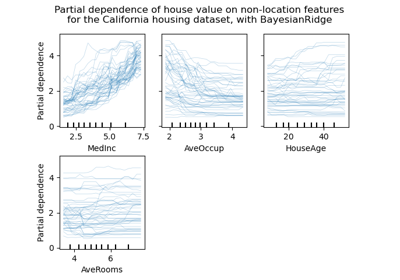Release Highlights for scikit-learn 0.24

Release Highlights for scikit-learn 0.24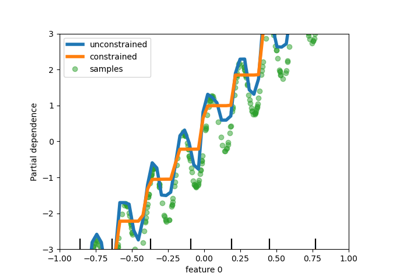Release Highlights for scikit-learn 0.23

Release Highlights for scikit-learn 0.23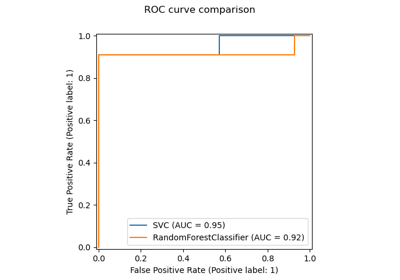Release Highlights for scikit-learn 0.22

Release Highlights for scikit-learn 0.22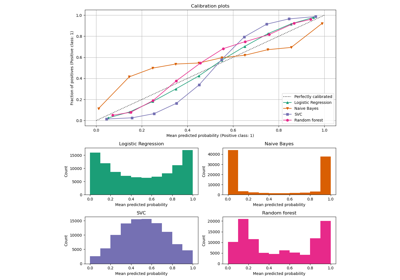Comparison of Calibration of Classifiers

Comparison of Calibration of Classifiers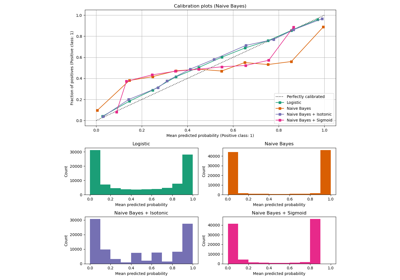Probability Calibration curves

Probability Calibration curves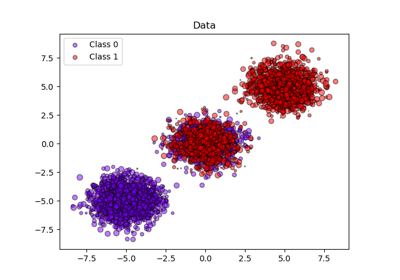Probability calibration of classifiers

Probability calibration of classifiers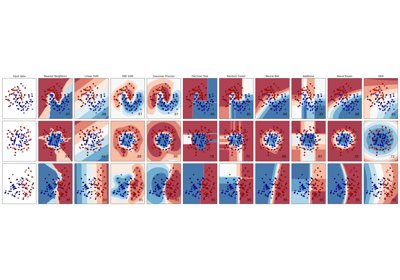Classifier comparison

Classifier comparisonRecognizing hand-written digits

Recognizing hand-written digits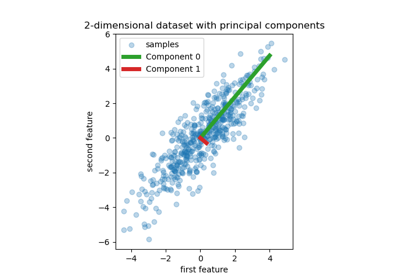Principal Component Regression vs Partial Least Squares Regression

Principal Component Regression vs Partial Least Squares Regression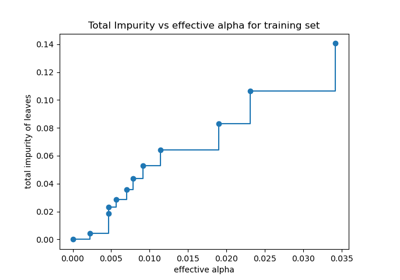Post pruning decision trees with cost complexity pruning

Post pruning decision trees with cost complexity pruning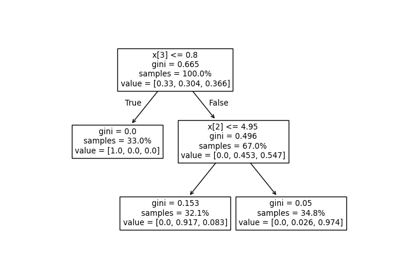Understanding the decision tree structure

Understanding the decision tree structure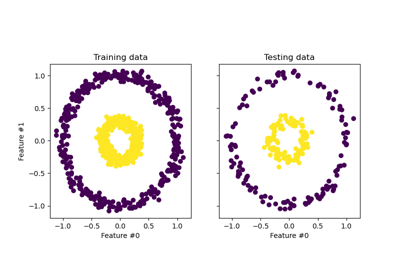Kernel PCA

Kernel PCA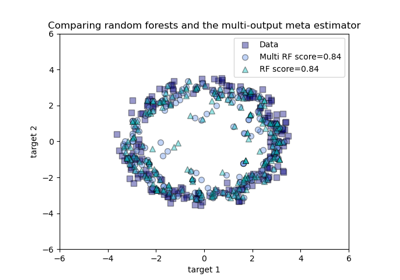Comparing random forests and the multi-output meta estimator

Comparing random forests and the multi-output meta estimator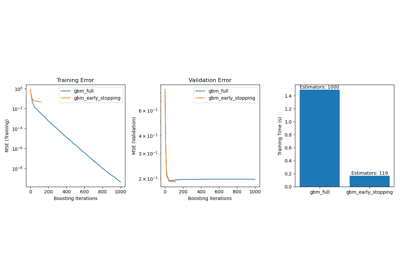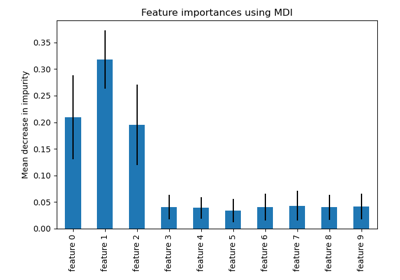Feature importances with a forest of trees

Feature importances with a forest of trees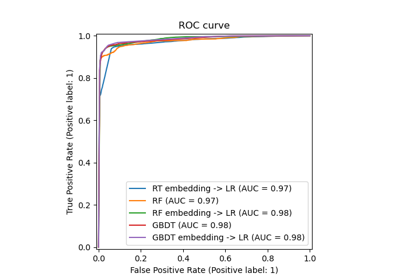Feature transformations with ensembles of trees

Feature transformations with ensembles of trees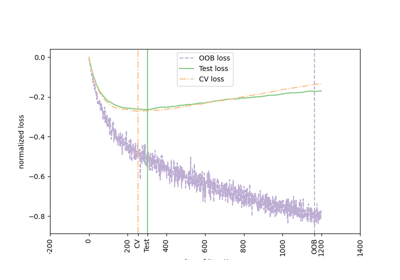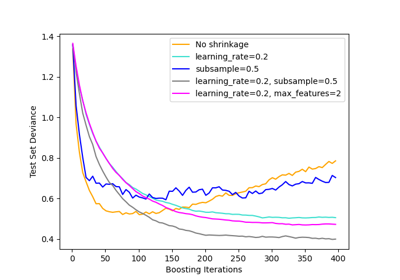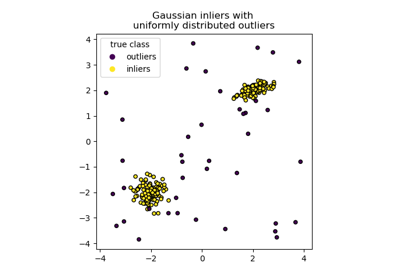IsolationForest example

IsolationForest example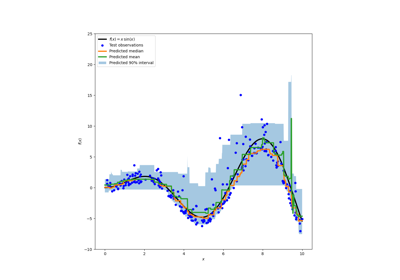Prediction Intervals for Gradient Boosting Regression

Prediction Intervals for Gradient Boosting Regression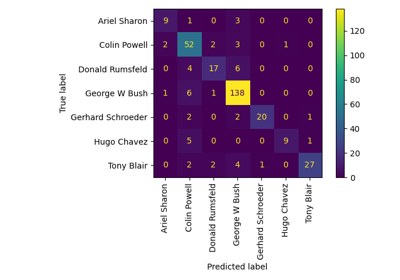Faces recognition example using eigenfaces and SVMs

Faces recognition example using eigenfaces and SVMs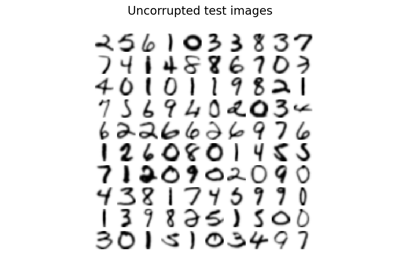Image denoising using kernel PCA

Image denoising using kernel PCA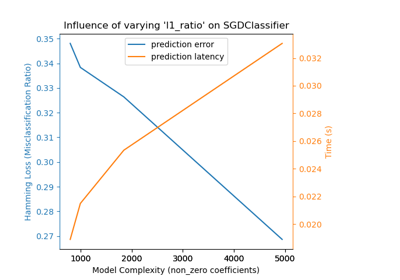Model Complexity Influence

Model Complexity Influence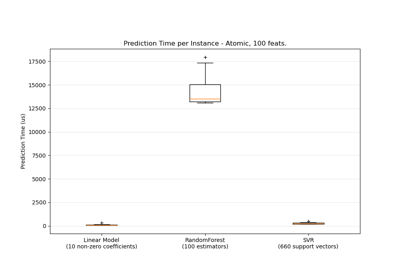Prediction Latency

Prediction LatencyPipeline ANOVA SVM

Pipeline ANOVA SVM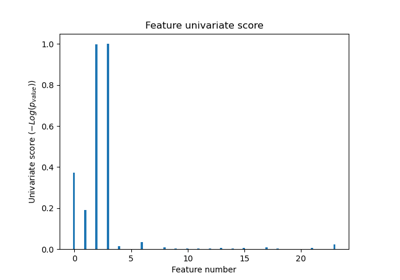Univariate Feature Selection

Univariate Feature SelectionComparing various online solvers

Comparing various online solvers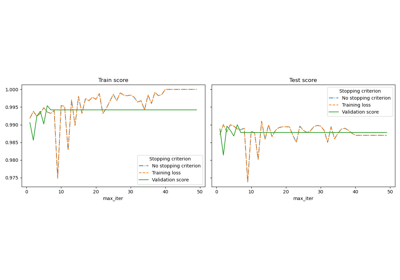Early stopping of Stochastic Gradient Descent

Early stopping of Stochastic Gradient DescentMNIST classification using multinomial logistic + L1

MNIST classification using multinomial logistic + L1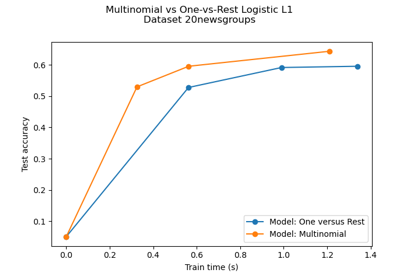Multiclass sparse logistic regression on 20newgroups

Multiclass sparse logistic regression on 20newgroups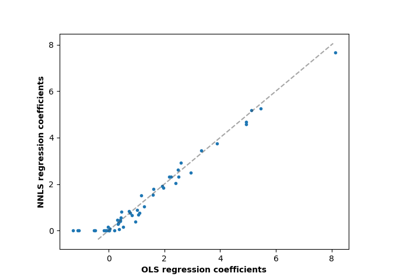Non-negative least squares

Non-negative least squares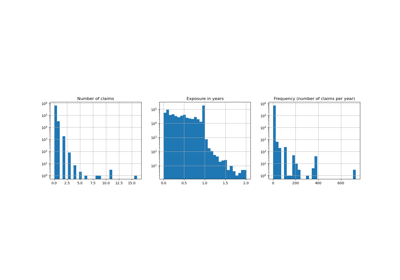Poisson regression and non-normal loss

Poisson regression and non-normal loss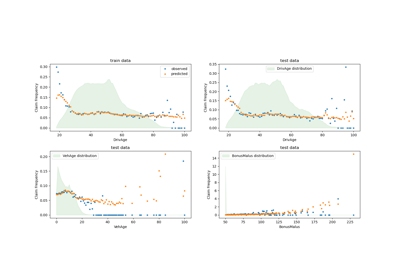Tweedie regression on insurance claims

Tweedie regression on insurance claims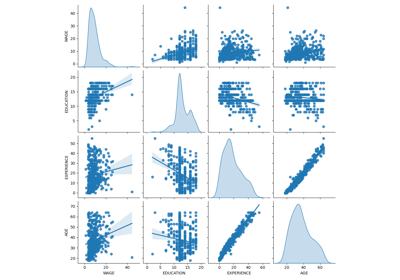Common pitfalls in the interpretation of coefficients of linear models

Common pitfalls in the interpretation of coefficients of linear models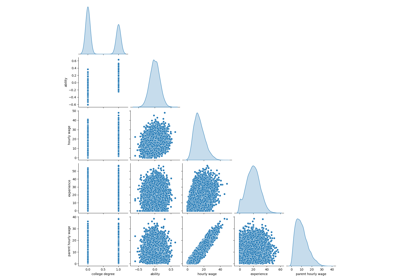Failure of Machine Learning to infer causal effects

Failure of Machine Learning to infer causal effects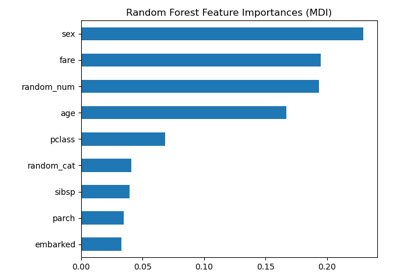Permutation Importance vs Random Forest Feature Importance (MDI)

Permutation Importance vs Random Forest Feature Importance (MDI)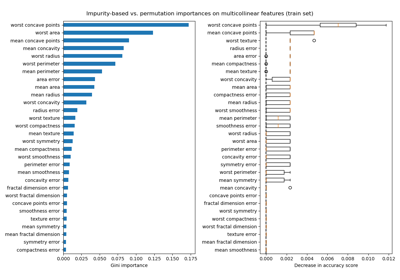Permutation Importance with Multicollinear or Correlated Features

Permutation Importance with Multicollinear or Correlated Features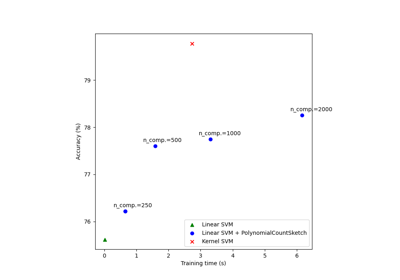Scalable learning with polynomial kernel approximation

Scalable learning with polynomial kernel approximation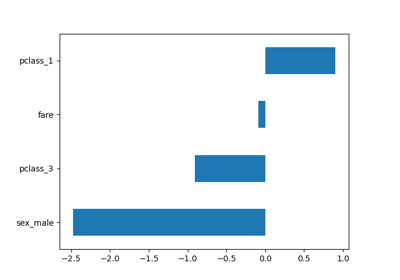Introducing the set_output API

Introducing the set_output API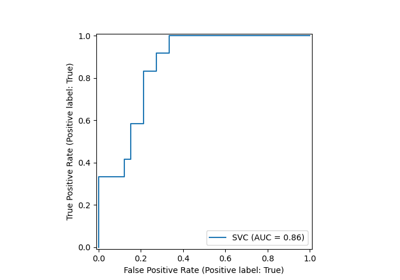ROC Curve with Visualization API

ROC Curve with Visualization API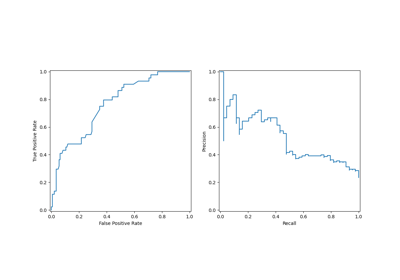Visualizations with Display Objects

Visualizations with Display Objects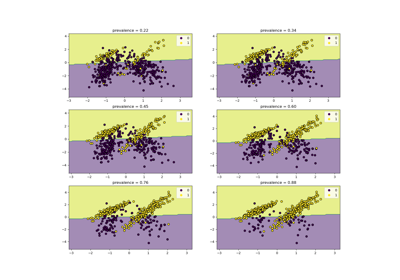Class Likelihood Ratios to measure classification performance

Class Likelihood Ratios to measure classification performance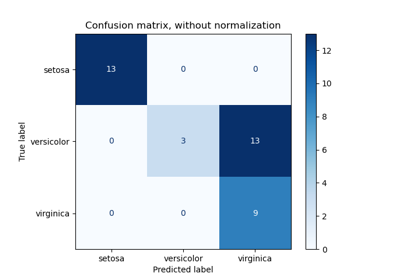Confusion matrix

Confusion matrixCustom refit strategy of a grid search with cross-validation

Custom refit strategy of a grid search with cross-validation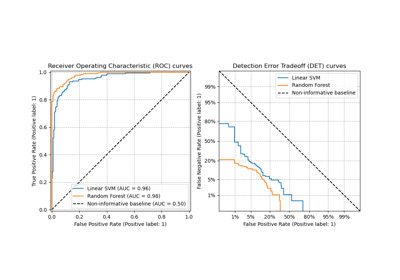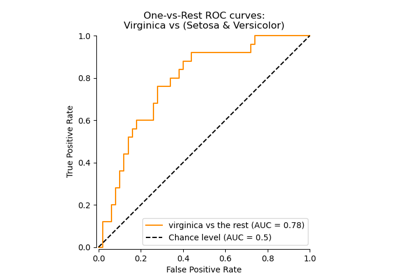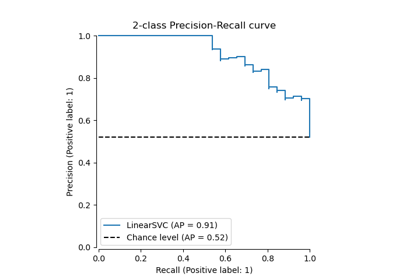Precision-Recall

Precision-Recall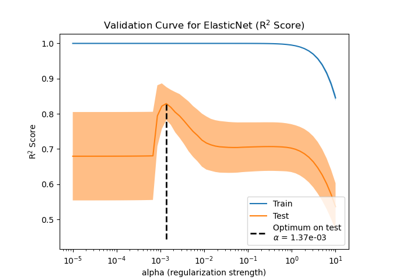Train error vs Test error

Train error vs Test error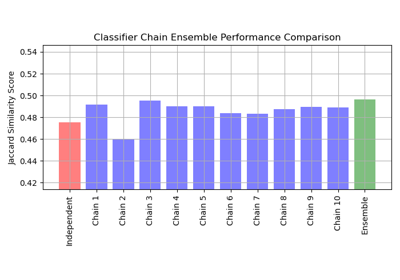Classifier Chain

Classifier Chain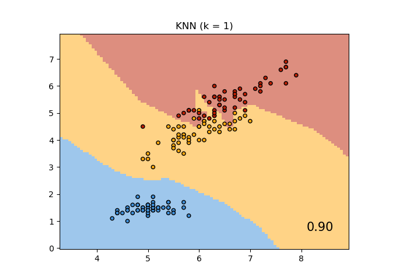Comparing Nearest Neighbors with and without Neighborhood Components Analysis

Comparing Nearest Neighbors with and without Neighborhood Components Analysis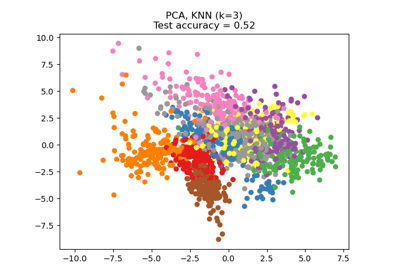Dimensionality Reduction with Neighborhood Components Analysis

Dimensionality Reduction with Neighborhood Components Analysis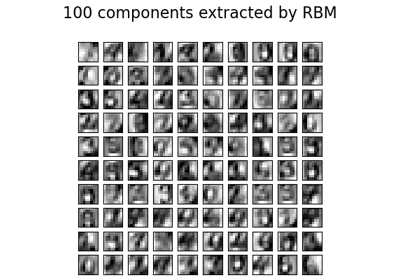Restricted Boltzmann Machine features for digit classification

Restricted Boltzmann Machine features for digit classification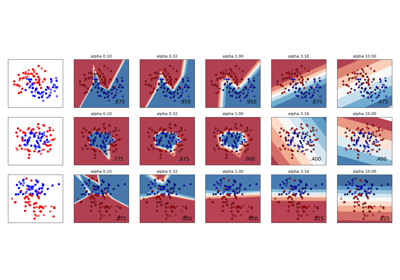Varying regularization in Multi-layer Perceptron

Varying regularization in Multi-layer Perceptron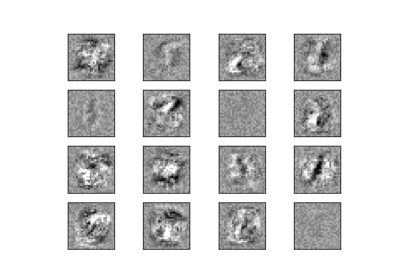Visualization of MLP weights on MNIST

Visualization of MLP weights on MNISTColumn Transformer with Mixed Types

Column Transformer with Mixed Types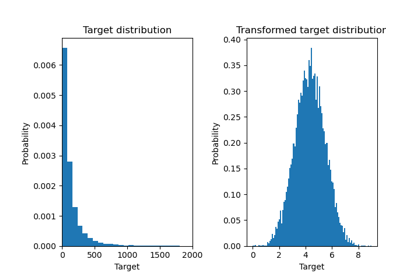Effect of transforming the targets in regression model

Effect of transforming the targets in regression model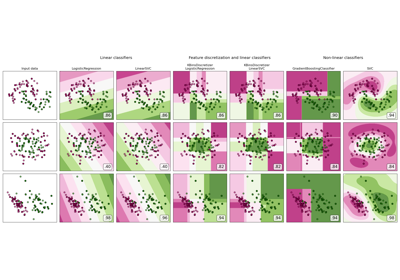Feature discretization

Feature discretization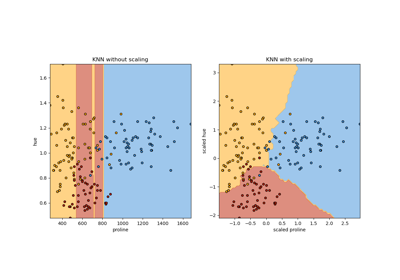Importance of Feature Scaling

Importance of Feature Scaling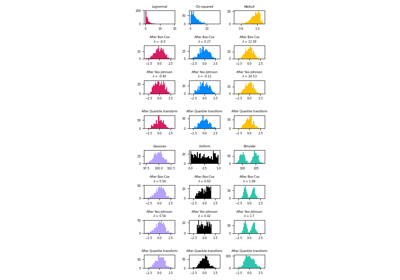Map data to a normal distribution

Map data to a normal distribution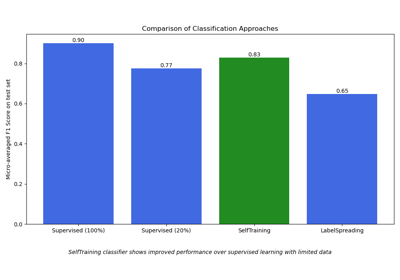Semi-supervised Classification on a Text Dataset

Semi-supervised Classification on a Text Dataset